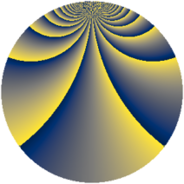# Properties

 Label 690.2.xLevel $690$ Weight $2$ Character orbit 690.x Rep. character $\chi_{690}(77,\cdot)$ Character field $\Q(\zeta_{44})$ Dimension $960$ Newform subspaces $1$ Sturm bound $288$ Trace bound $0$

# Related objects

## Defining parameters

 Level: $$N$$ $$=$$ $$690 = 2 \cdot 3 \cdot 5 \cdot 23$$ Weight: $$k$$ $$=$$ $$2$$ Character orbit: $$[\chi]$$ $$=$$ 690.x (of order $$44$$ and degree $$20$$) Character conductor: $$\operatorname{cond}(\chi)$$ $$=$$ $$345$$ Character field: $$\Q(\zeta_{44})$$ Newform subspaces: $$1$$ Sturm bound: $$288$$ Trace bound: $$0$$

## Dimensions

The following table gives the dimensions of various subspaces of $$M_{2}(690, [\chi])$$.

Total New Old
Modular forms 3040 960 2080
Cusp forms 2720 960 1760
Eisenstein series 320 0 320

## Trace form

 $$960q + 8q^{3} + 8q^{6} + O(q^{10})$$ $$960q + 8q^{3} + 8q^{6} - 8q^{12} - 16q^{13} + 8q^{15} + 96q^{16} + 72q^{18} + 16q^{22} + 32q^{25} + 8q^{27} - 16q^{31} + 36q^{33} - 8q^{36} + 24q^{37} - 48q^{43} - 16q^{46} - 8q^{48} - 32q^{51} + 16q^{52} - 64q^{55} - 16q^{57} + 8q^{60} - 96q^{61} + 72q^{63} - 144q^{66} + 64q^{67} - 16q^{70} + 16q^{72} + 48q^{73} + 4q^{75} - 24q^{78} - 248q^{81} - 32q^{82} + 64q^{85} - 8q^{87} + 16q^{88} + 40q^{90} - 96q^{91} - 104q^{93} - 8q^{96} - 264q^{97} + O(q^{100})$$

## Decomposition of $$S_{2}^{\mathrm{new}}(690, [\chi])$$ into newform subspaces

Label Dim. $$A$$ Field CM Traces $q$-expansion
$$a_2$$ $$a_3$$ $$a_5$$ $$a_7$$
690.2.x.a $$960$$ $$5.510$$ None $$0$$ $$8$$ $$0$$ $$0$$

## Decomposition of $$S_{2}^{\mathrm{old}}(690, [\chi])$$ into lower level spaces

$$S_{2}^{\mathrm{old}}(690, [\chi]) \cong$$ $$S_{2}^{\mathrm{new}}(345, [\chi])$$$$^{\oplus 2}$$